# Test: Introduction Of Inverse Trigonometry

## 10 Questions MCQ Test Mathematics (Maths) Class 12 | Test: Introduction Of Inverse Trigonometry

Description
Attempt Test: Introduction Of Inverse Trigonometry | 10 questions in 10 minutes | Mock test for JEE preparation | Free important questions MCQ to study Mathematics (Maths) Class 12 for JEE Exam | Download free PDF with solutions
QUESTION: 1

### The value of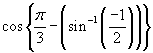is given by​

Solution: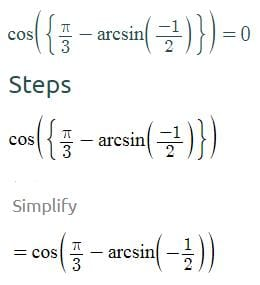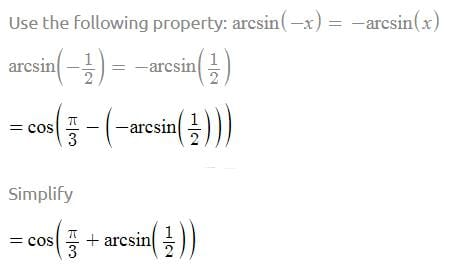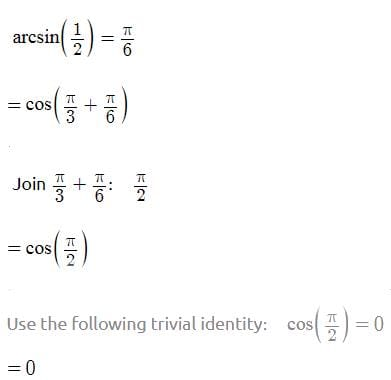QUESTION: 2

### The principal value of tan-1 (-1) is given by​:

Solution:

Let y = tan-1(-1)

tan y = -1

tan y = tan(-π/4)

Range of principal value of tan-1 is
(-π/2 , π/2)

SInce - 1 is negative,
the principle value of tan-1(-1) is - π/4​

QUESTION: 3

### if 5 sin θ = 3, then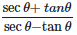is equal to

Solution:

sin θ is 3/5.
on simplifying:
(secθ + tanθ)/(secθ - tanθ)
We get, (1+sin θ)/(1-sin θ)
=(1+3/5)/(1-3/5)
=(8/2)
=4

QUESTION: 4

Value of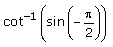Solution:

Since sin-1(-π/2) = -sin-1(π/2) = -1

so, cot -1(-1)

let (- cot-11) = α

cot α = -1 = cot (π/2+π/4) = 3π/4

QUESTION: 5

What is the solution of cot⁡(sin-1⁡x)?

Solution:

Let sin-1⁡x = y.
From ∆ABC, we get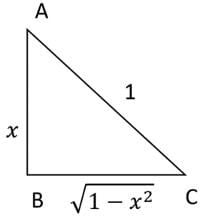y = sin-1⁡x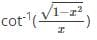∴cot⁡(sin-1⁡x)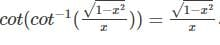QUESTION: 6

Find the value of sin-1⁡(3/5) + sin-1⁡(4/5) + cos-1⁡(√3/2).

Solution:

Using the formula sin-1⁡x + sin-1⁡y
=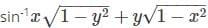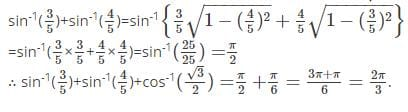QUESTION: 7

If x< 0 then value of tan-1(x) + tan-1 (1/x) is equal to

Solution:

We know that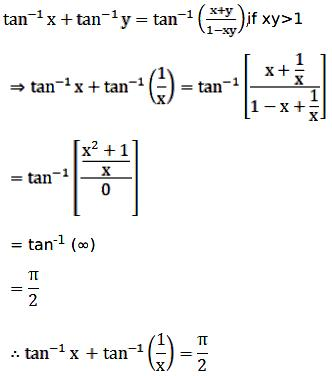QUESTION: 8

Find the value of tan-1⁡(1/3) + tan-1⁡(1/5) + tan-1⁡(1/7).

Solution: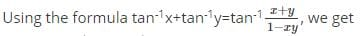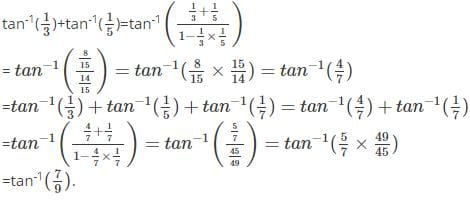QUESTION: 9

Evaluate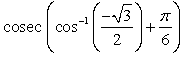Solution: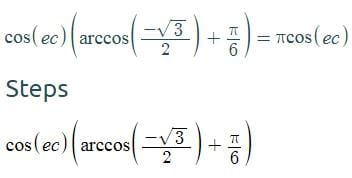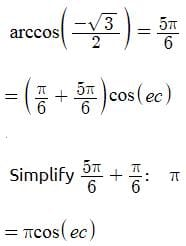QUESTION: 10

What is the value of 2 tan-1⁡x?

Solution:

Let 2 tan-1⁡x = y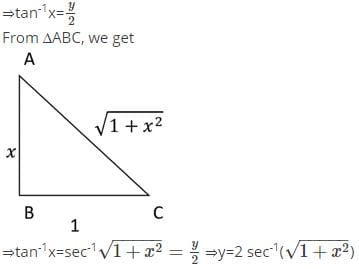Use Code STAYHOME200 and get INR 200 additional OFF Use Coupon Code